### Measure

Based on the selection type, calculates and displays either area statistics, line lengths and angles, or point coordinates. Area statistics are calculated if there is no selection or if a subregion of the image has been selected using one of the first four tools in the tool bar. Calculates line length and angle if a line selection has been created using one of the three line selection tools. Records coordinates if one or more points have been defined using the point selection tool. Use the Analyze>Set Measurements command to specify what area statistics are recorded.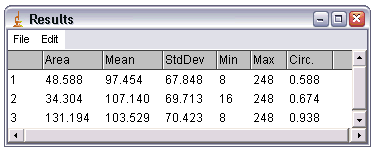With RGB images, results are calculated using brightness values. RGB pixels are converted to brightness values using the formula V=(R+G+B)/3, or V=0.299R+0.587G+0.114B if "Weighted RGB Conversions" is checked in Edit>Option>Conversions. The default weighting factors are the ones used to convert to from RGB to YUV, the color encoding system used for analog television. The weighting factors can be changed using the setRGBWeights macro function.

With line selections, the following parameters can be recorded: length, angle (straight lines only), mean, standard deviation, mode, min, max and bounding rectangle (v1.34l or later). The mean, standard deviation, etc. are calculated from the values of the pixels along the line.

To export the measurements as a tab-delimited text file, select File>Save As>Measurements from the ImageJ menu bar or File>Save As from the "Results" window menu bar. Copy the measurements to the clipboard by selecting Edit>Copy All from the "Results" window menu bar. You can also save measurements by right-clicking in the Results window and selecting Save As or Copy All from the popup menu.

The width of the columns in the "Results" window can be adjusted by clicking on and dragging the vertical lines that separate the column headings.

### Analyze Particles...

This command counts and measures objects in binary or thresholded images. It works by scanning the image or selection until it finds the edge of an object. It then outlines the object using the wand tool, measures it using the Measure command, fills it to make it invisible, then resumes scanning until it reaches the end of the image or selection. Press the esc key to abort this process. Use Image>Adjust>Threshold to threshold an image.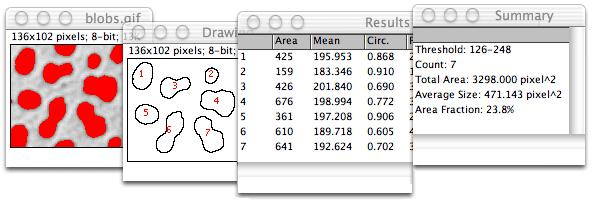Use the dialog box to configure the particle analyzer. Particles outside the range specified in the Size field are ignored. Enter a single value in Size and particles smaller than that value are ignored. Particles with circularity values outside the range specified in the Circularity field are also ignored. The formula for circularity is 4pi(area/perimeter^2). A value of 1.0 indicates a perfect circle.Select Outlines from the "Show:" popup menu and ImageJ will open a window containing numbered outlines of the measured particles. Select Masks to display filled outlines of the measured particles or Ellipses to display the best fit ellipse of each measured particles.Check Display results to have the measurements for each particle displayed in the "Results" window. Check Clear Results to erase any previous measurement results. Check Summarize to display, in a separate window, the particle count, total particle area, average particle size, and area fraction. Check Exclude on Edges to ignore particles touching the edge of the image or selection.

Check Include Holes to include interior holes. Disable this option to exclude interior holes and to measure particles enclosed by other particles. When this option is enabled, ImageJ finds the extent of each particle by tracing the outer edge. When it is disabled, ImageJ finds the extent by flood filling.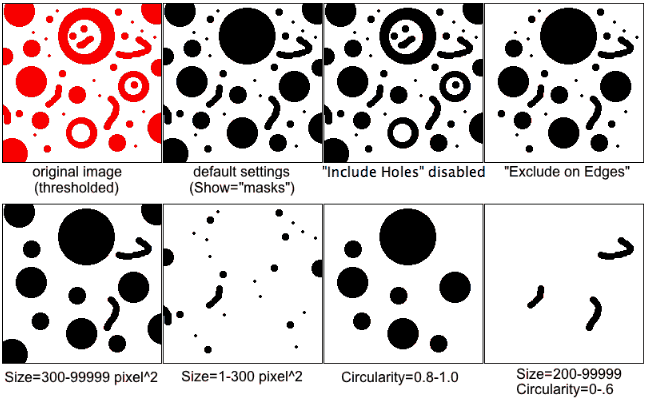The Record Starts option allows plugins and macros to recreate particle outlines using the doWand(x,y) function. The CircularParticles macro demonstrates how to use this feature.

Check Add to Manager and the measured particles will be added to the ROI Manager.

### Summarize

For each column in the results table, calculates and displays the mean, standard deviation, minimum and maximum of the values in that column.

### Clear Results

Erases the results table and resets the measurement counter.

### Set Measurements...

Use this dialog box to specify which measurements are recorded by Analyze>Measure and Analyze>Analyze Particles.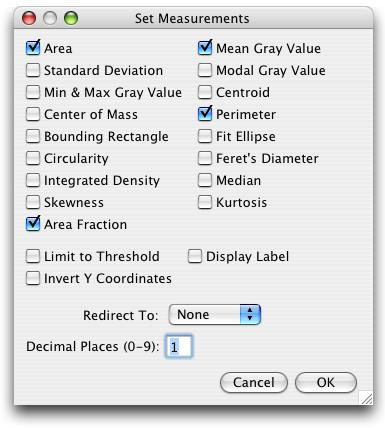Area - Area of selection in square pixels. Area is in calibrated units, such as square millimeters, if Analyze>Set Scale was used to spatially calibrate the image.

Mean Gray Value - Average gray value within the selection. This is the sum of the gray values of all the pixels in the selection divided by the number of pixels. Reported in calibrated units (e.g., optical density) if Analyze>Calibrate was used to calibrate the image. For RGB images, the mean is calulated by converting each pixel to grayscale using the formula gray=0.299red+0.587green+0.114blue or the formula gray=(red+green+blue)/3 if "Unweighted RGB to Grayscale Conversion" is checked in Edit>Options>Conversions.

Standard Deviation - Standard deviation of the gray values used to generate the mean gray value.

Modal Gray Value - Most frequently occurring gray value within the selection. Corresponds to the highest peak in the histogram.

Min & Max Gray Level - Minimum and maximum gray values within the selection.

Centroid - The center point of the selection. This is the average of the x and y coordinates of all of the pixels in the image or selection. Uses the X and Y Results table headings.

Center of Mass - This is the brightness-weighted average of the x and y coordinates all pixels in the image or selection. Uses the XM and YM headings. These coordinates are the first order spatial moments.

Perimeter - The length of the outside boundary of the selection.

Bounding Rectangle - The smallest rectangle enclosing the selection. Uses the headings BX, BY, Width and Height, where BX and BY are the coordinates of the upper left corner of the rectangle.

Fit Ellipse - Fit an ellipse to the selection. Uses the headings Major, Minor and Angle. Major and Minor are the primary and seconday axis of the best fitting ellipse. Angle (0-180 degrees) is the angle between the primary axis and a line parallel to the x-axis of the image. The coordinates of the center of the ellipse are displayed as X and Y if Centroid is checked. Note that ImageJ cannot calculate the major and minor axis lengths if Pixel Aspect Ratio in the Set Scale dialog is not 1.0.

There are several ways to view the ellipse. The Edit>Selection>Fit Ellipse command replaces an area selection with the best fit ellipse. The DrawEllipse macro draws (destructively) the best fit ellipse and the major and minor axis. Select "Ellipses" from the Show: drop down menu in the particle analyzer and it will draw the ellipse for each particle in a separate window.

Shape Descriptors (previously Circularity) - Calculate and display the following shape descriptors:

Circ. (circularity): 4π*area/perimeter^2. A value of 1.0 indicates a perfect circle. As the value approaches 0.0, it indicates an increasingly elongated shape. Values may not be valid for very small particles.

AR (aspect ratio): major_axis/minor_axis. Enable "Fit Ellipse" in Analyze>Set Measurements to have the major and minor axis displayed.

Round (roundness): 4*area/(π*major_axis^2), or the inverse of the aspect ratio.

Solidity: area/convex area. The Edit>Selection>Convex Hull command makes an area selection convex.

Feret's Diameter - The longest distance between any two points along the selection boundary, also known as maximum caliper. Uses the Feret heading. FeretAngle (0-180 degrees) is the angle between the Feret's diameter and a line parallel to the x-axis of the image. MinFeret is the minimum caliper diameter. The starting coordinates of the Feret's diameter (FeretX and FeretY) are also displayed. The DrawFeretDiameter macro draws the Feret's diameter of the current selection.

Integrated Density - Calculates and displays two values: "IntDen" (the product of Area and Mean Gray Value) and "RawIntDen" (the sum of the values of the pixels in the image or selection). "RawIntDen" is only available in ImageJ 1.44c or later. "IntDen" and "RawIntDen" values are the same for uncalibrated image. The Dot Blot Analysis example demonstrates how to use this option to analyze a dot blot assay.

Median - The median value of the pixels in the image or selection.

Skewness - The third order moment about the mean. The documentation for the Moment Calculator plugin explains how to interpret spatial moments.

Kurtosis - The fourth order moment about the mean.

Area Fraction - The percentage of pixels in the image or selection that have been highlighted in red using Image>Adjust>Threshold. For non-thresholded images, the percentage of non-zero pixels.

Stack Position - The current position (channel, slice and frame) in the stack or hyperstack. Uses the headings "Ch", "Slice" and "Frame".

Limit to Threshold - If checked, only thresholded pixels are included in measurement calculations. Use Image>Adjust>Threshold to set the threshold limits.

Display Label - If checked, the image name and slice number (for stacks) are recoded in the first column of the results table.

Invert Y Coordinates - If checked, the XY origin is assumed to be the lower left corner of the image window instead of the upper left corner.

Redirect To - The image selected from this popup menu will be used as the target for statistical calculations done by the Measure and Analyze Particles commands. The Redirect To feature allows you to outline a structure on one image and measure the intensity of the corresponding region in another image. With ImageJ 1.35d or later this feature also works with stacks. Note that it is the thresholding of the target image that is used when Limit to Threshold is enabled.

Decimal Places - This is the number of digits to the right of the decimal point in real numbers displayed in the results table and in histogram windows.

### Set Scale...

Use this dialog to define the spatial scale of the active image so measurement results can be presented in calibrated units, such as millimeters. Before using this command, use the straight line selection tool to make a line selection that corresponds to known distance. Then, bring up the Set Scale dialog, enter the known distance and unit of measurement, then click OK. ImageJ will have automatically filled in the Distance in Pixels field based on the length of the line selection.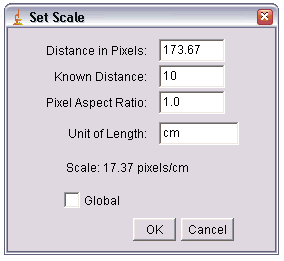Set Distance in Pixels to zero to revert to pixel measurements.

Setting Pixel Aspect Ratio to a value other than 1.0 enables support for different horizontal and vertical spatial scales, for example 100 pixels/cm horizontally and 95 pixels/cm vertically. To set the pixel aspect ratio, measure the width and height (in pixels) of a digitized object with a known 1:1 aspect ratio. Enter the measured width (in pixels) in Distance in Pixels. Enter the known width in Known Distance. Then calculate the aspect ratio by dividing the width by the height and enter it in Pixel Aspect Ratio.

When Global is checked, the scale defined in this dialog is used for all images instead of just the active image.

Three tutorials are available that show how to use the Set Scale command: Area Measurements (PDF), DNA Contour Length Measurement and Spatial Calibration (Fiji).

### Calibrate...

Use this dialog box to calibrate an image to a set of density standards, for example radioactive isotope standards or a calibrated optical density step tablet. Before using this command, use Analyze>Clear Results to reset the measurement counter and use one of the selection tools and Analyze>Measure to record the mean gray value of each of the standards. There is an example that shows how to calibrate to an optical density step tablet.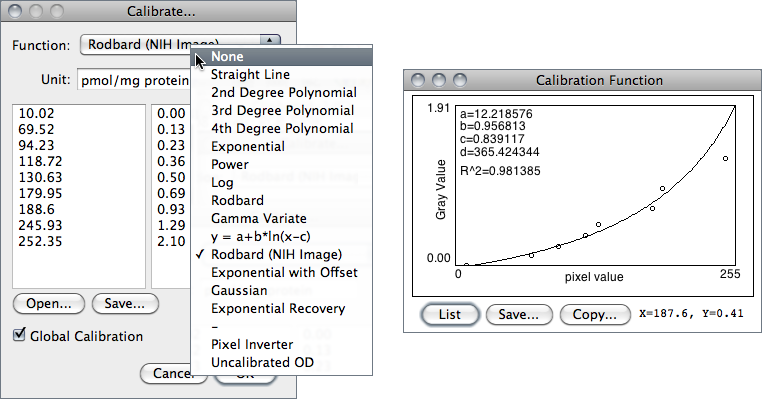When finished making the measurements, select Analyze>Calibrate to display the Calibrate dialog box. To calibrate the image, enter the known standard values in the right column, select a curve fitting method from the popup menu, enter the unit of measurement, and click OK. ImageJ will then display the calibration function. If the function is not satisfactory, bring up the Calibrate dialog box again and select a different curve fitting method.

Rodbard is a four parameter general curve fit function proposed by David Rodbard at NIH. The form of the equation is:

y = d + (a - d) / (1 + (x/c)^b)
Selecting Uncalibrated OD from the popup menu causes ImageJ to convert gray values to uncalibrated optical density values using the function
Uncalibrated OD = log10(255 / PixelValue)
You do not need to measure OD standards or enter known OD values to enable this feature.

### Histogram

Calculates and displays a histogram of the distribution of gray values in the active image or selection. The x-axis represents the possible gray values and the y-axis shows the number of pixels found for each gray value. The total pixel count is also calculated and displayed, as well as the mean, modal, minimum and maximum gray value.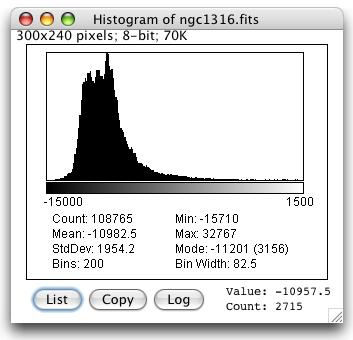Use the List or Copy buttons to save the histogram data. Click on Log to display a log-scaled version of the histogram. The number to the right of Value:, which changes as you move the cursor, is the grayscale value corresponding to the x-axis cursor position and Count: is the number of pixels that have that value.

The getHistogram() macro function can be used to get the "value" and "count" data displayed when you click the List button:

```   getHistogram(values, counts, 256);
for (i=0; i<values.length; i++)
print(values[i], counts[i]);
```
With stack histograms, use the Plot.getValues() macro function to get the data:
```   requires("1.41k");
run("Histogram", "stack");
Plot.getValues(values, counts);
for (i=0; i<values.length; i++)
print(values[i], counts[i]);
```
With RGB images, the histogram is calculated by converting each pixel to grayscale using the formula gray=0.299red+0.587green+0.114blue or the formula gray=(red+green+blue)/3 if "Unweighted RGB to Grayscale Conversion" is checked in Edit/Options/Conversions. With 16-bit images, the range of gray values between the Min and Max values is divided into 256 bins. With 32-bit images, the number of bins is specified in this dialog box: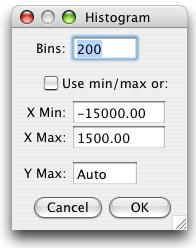Check Use min/max and the x-axis range is determined by the minimum and maximum values in the image or selection, or specify X Min and X Max values to fix the x-axes range. Enter a Y Max value to fix the y-axis range or enter "Auto" to have the range determined by the largest bin count. In ImageJ 1.35a or later, hold down the alt key (or press alt-h) to use this dialog with 8-bit, 16-bit and RGB images.

### Plot Profile

Displays a two-dimensional graph of the intensities of pixels along a line within the image. The x-axis represents distance along the line and the y-axis is the pixel intensity.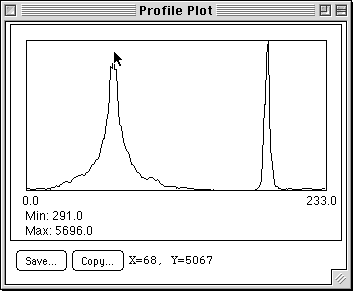For rectangular selections, displays a "column average plot", where the x-axis represents the horizontal distance through the selection and the y-axis the vertically averaged pixel intensity. To average horizontally, hold down the alt key. Use the Save or Copy buttons to save the profile data, formatted as a sequence of lines each containing a single number.

The Dynamic Profiler plugin creates a profile plot that is continuously updated as the selection is moved or the image is updated. The StackProfilePlot macro generates profile plots of all the images in a stack and saves them in another stack.

### Surface Plot

Displays a three-dimensional graph of the intensities of pixels in a grayscale or pseudo color image. Creates a stack of plots when the source is a stack. Some plots can be improved by adjusting the contrast of the source image or smoothing it. When plotting a stack, closing the plot stack window will abort the plotting process.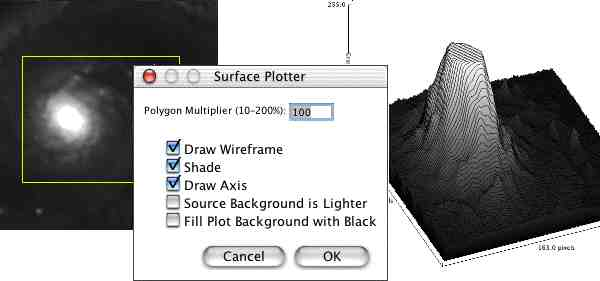Change the Polygon Multiplier to adjust the number of profiles used to generate the plot. Check Draw Wireframe to have the outline each profile drawn in black. Check Shade to generate a shaded plot. The plot will be in color if the source image uses a color LUT. Check Draw Axis to have the three axis drawn and labeled. If Source Background is Lighter is checked, lighter areas in the source image represent lower elevations (valleys) while darker areas in the source image represent higher elevations (peaks). If Fill Plot Background with Black is checked, the plot is drawn with a black background.

Kai Uwe Barthel's Interactive 3D Surface Plot plugin also generates surface plots. It works with all image types and you can interactively adjust viewing angle, perspective, scale, lighting and smoothing.

Use the commands in this submenu to analyze one-dimensional electrophoretic gels. These commands are similar to the Gel Plotting Macros distributed with NIH Image. Both use a simple graphical method that involves generating lane profile plots, drawing lines to enclose peaks of interest, and then measuring peak areas using the wand tool.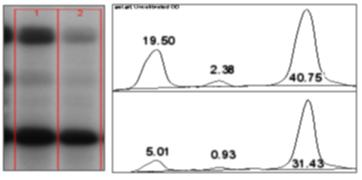Here is what you need to do to analyse a 1-D gel:

1. Use the rectangular selection tool to outline the first lane. This should be the left most lane if the lanes are vertical or the top lane if the lanes are horizontal. Note that lanes are assummed to be vertical unless the width of the initial selection is at least twice its height.
2. Select Analyze>Gels>Select First Lane (or press "1") and the lane will be outlined and "Lane 1 selected" displayed in the status bar.
3. Move the rectangular selection right to the next lane (or down if the lanes are horizontal) and select Analyze>Gels>Select Next Lane (or press "2"). The selected lane is outlined and labeled, and "Lane n selected" is displayed in the status bar.
4. Repeat the previous step for each remaining lane.
5. Select Analyze>Gels>Plot Lanes (or press "3") to generate the lane profile plots.
6. Use the straight line selection tool to draw base lines and/or drop lines so that each peak of interest defines a closed area. To get to all the lanes, it may be necessary to scroll the image vertically using the "Hand" tool. (Hold down the space bar to temporarily switch to this tool).
7. For each peak, measure the size by clicking inside with the wand tool. If necessary, scroll the image vertically by holding down the space bar and dragging.
8. Select Analyze>Gels>Label Peaks to label each measured peak with its size as a percent of the total size of the measured peaks.
For practice, a sample gel is available in the File>Sample Images submenu. A tutorial is available that shows how to use this procedure to analyze a dot blot. There is another tutorial at www.lukemiller.org.

### Save XY Coordinates...

Writes to a text file the XY coordinates and pixel value of all non-background pixels in the active image. Background is assumed to be the value of the pixel at the upper left corner of the image. For grayscale images, writes three values per line (x, y, and value), separated by spaces. For RGB images, writes five values per line (x, y, red, green and blue). The origin of the coordinate system is at the lower left corner of the image.

### Fractal Box Count...

Counts the number of boxes of an increasing size needed to cover a one pixel binary object boundary. The box size and the number of boxes necessary to cover the boundary are plotted on a log-log plot and the fractal dimension determined from the slope, i.e. D=-slope. For more information, see the source code (FractalBoxCounter.java).

### Analyze Line Graph

ImageJ can be used to recover numeric coordinate data from scanned line graphs using the following procedure. Steps 1-6 are not necessary for binary (black and white) graphs. For practice, use the File>Open Samples>Line Graph sample image.
1. Open the image containing the graph.
2. Open the thresolding tool (shift-t).
3. Adjust the threshold so the graph is highlighted in red.
4. Click on "Apply" (make sure foreground is black and background is white).
5. Close the thresholding tool.
6. Use the oval selection tool as an erasor (press backspace to erase) to isolate a single curve (note: background color must be white).
7. Select the curve by clicking to the left of it with the wand tool.
8. Use Edit/>Clear Outside to erase everything but the curve.
9. Use Analyze>Tools>Analyze Line Graph get the XY coordinates.

### ROI Manager

The ROI (Region of Interest) Manager is a tool for working with multiple selections. The selections can be from different locations on an image or from different slices of a stack. All selection types, including points and lines, are supported.Click Add to add the current selection to the list, or press "t", the keyboard shortcut for the Edit>Selection>Add to Manager command. The Roi manager creates a three part label. The first part (stacks only) is the slice number, the second is the Y-coordinate of the selection and the third is the X-coordinate. Click on a label to restore the associated selection to the current image. With stacks, the selection is restored to the slice it came from. Click on Show All to display all the selections on the list. Hold down the shift key while clicking Add to "add and draw" and the alt key to "add and rename".

Install the ROIManagerMacros macro set and you will be able to add a selection by pressing the "1" key, add and name by pressing "2", add and draw by pressing "3", and add and advance to the next slice by pressing "4".

Update replaces the selected ROI on the list with the current selection. This is usually a modified version of a selection from the ROI Manager list.

Delete deletes the selected ROIs from the list. Deletes all the ROIs if none are selected.

Use Rename to rename the selected ROI.

Open opens a ".roi" file and adds it to the list or opens a ZIP archive (".zip" file) and adds all the selections contained in it to the list. Use the Open All macro to add all the ".roi" files in a folder.

Save saves the selected ROI as an ".roi" file. If no ROIs are selected, saves all the ROI Manager selections as a ZIP archive.

Measure measures the selected ROIs, or if none are selected, all ROIs on the list. Use Analyze>Set Measurements to specify the parameters to measure. With a stack, you will be given the option to measure all the slices if all ROIs are associated with the first slice (have labels like 0001-xxxx-yyyy) or all have labels in the form xxxx-yyyy.

If this is not the case, use the More>>Multi Measure function. Multi Measure, based on Bob Dougherty's Multi_Measure plugin, measures all the ROIs on all the images in the stack, creating a Results table with either one row per image or one row per measurement.

Deselect deselects any selected ROIs on the list. Delete, Save, Measure, More>>Draw, More>>Fill, More>>Label and More>>Combine work with all ROIs on the list when none are selected.

Show All displays a non-destructive overlay that outlines and labels all ROI Manager selections. Click Show All a second time to remove the overlay. Click on a label (selection number) in the overlay to activate the corresponding selection. That selection can then be moved or editied and the overlay will be dynamically updated. The color and behavior of the "Show All" overlay can be changed in the More>>Options dialog box.

Draw draws outline of the selected ROIs using the current foreground color and line width. Outlines all selections on the list if none are selected. Click in the Image>Color>Color Picker window to change the foreground color. Use the Edit>Options>Line Width command to set the line width.

Fill fills the selected ROIs using the current foreground color. Fills all selections on the list if none are selected. Click in the Image>Color>Color Picker window to change the foreground color.

Label labels and outlines the selected ROIs using the current foreground color. Labels and outlines all selections on the list if none are selected. Unlike Show All, this changes the image contents.

Combine uses the union operator on the selected ROIs to create a composite selection. Combines all the ROIs if none are selected.

Split splits the current selection (it must be a composite selection) into its component parts and adds them to the ROI Manager.

Add Particles adds objects segmented by the particle analyzer to the ROI Manager. Requires that "Record Starts" be checked in the Analyze>Analyze Particles dialog box. Particle analyzer objects can also be added to the ROI Manager by checking "Add to Manager" in the Analyze Particles dialog box.

Multi Measure, (based on Bob Dougherty's Multi_Measure plugin) measures all the ROIs on all images in the stack, creating a Results table with either one row per image or one row per measurement.

Sort sorts the list in alphanumeric order.

Specify lets you specify an ROI in the same way as the Edit>Selection>Specify command.

Remove Slice Info removes the information in the ROI names that associates them with particular stack slices.